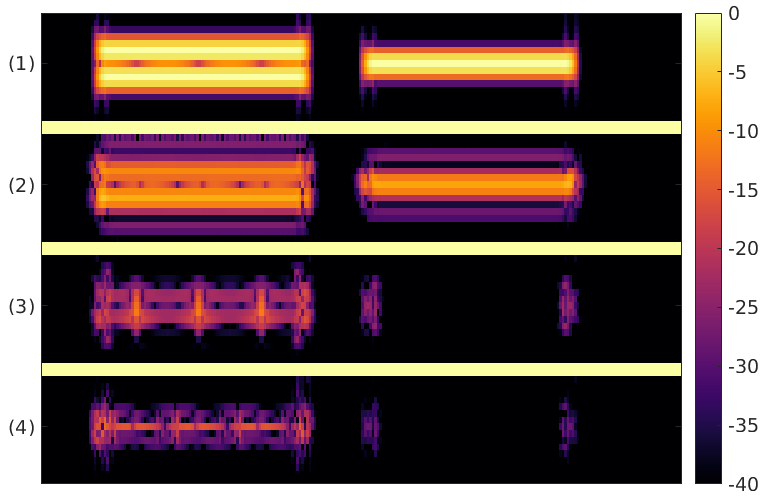• Original
• TFGAN-M
• TFGAN-MTF
• WaveGAN
• Original
• TFGAN-M
• WaveGAN
• Commands
• PianoIt may seem straightforward to restore the phase from its time-direction derivative by summation along frequency channels as proposed by Engel et Al. Even on real, unmodified STFTs, the resulting phase misalignment introduces cancellation between frequency bands resulting in energy loss, see Figure above (2) for a simple example. In practice, such cancellations often leads to clearly perceptible changes of timbre (see below). Moreover, in areas of small STFT magnitude, the phase is known to be unreliable (see Balazs et Al) and highly sensitive to distortions (see Alaifari et Al), such that it cannot be reliably modelled and synthesis from generated phase derivatives is likely to introduce more distortion. Phase-gradient heap integration (PGHI) (see Prusa et Al) relies on the phase-magnitude relations and bypasses phase instabilities by avoiding integration through areas of small magnitude, leading to significantly better and more robust phase estimates see Figure above (4). PGHI often outperforms more expensive, iterative schemes relying on alternate projection, e.g., Griffin-Lim, at the phaseless reconstruction (PLR) task. The following examples were computed on samples from the EBU SQAM dataset.
• Original
• Phase from the time-direction derivative
• Phase from PGHI
• Original (2)
• Phase from the time-direction derivative (2)
• Phase from PGHI (2)